The Bead Unbaffled - An Abacus Manual

###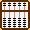Short Division Techniques - Chinese Suan Pan

This is a Chinese technique for doing short division. This technique works best with problems where divisors are made up of whole numbers and have a value of less than 10. Before going on to try traditional Chinese long division techniques, it's a good idea to completely understand how this short division method works. After a little practice many find this technique enhances their ability to solve problems of long division using division techniques as taught by Takashi Kojima.

Below is a list of traditional rules also known as the Chinese Division Table. Understanding these rules is integral to understanding this method. They may look a little complicated at first glance but they are easily understood. As such there should be no need to commit them to memory. (See below for a brief explanation.)

 Chinese Division Table 1/1 = forward 1 1/2 = 5 2/2 = forward 1 1/3 = 3 plus 1 2/3 = 6 plus 2 3/3 = forward 1 1/4 = 2 plus 2 2/4 = 5 3/4 = 7 plus 2 4/4 = forward 1 1/5 = 2 2/5 = 4 3/5 = 6 4/5 = 8 5/5 = forward 1 1/6 = 1 plus 4 2/6 = 3 plus 2 3/6 = 5 4/6 = 6 plus 4 5/6 = 8 plus 2 6/6 = forward 1 1/7 = 1 plus 3 2/7 = 2 plus 6 3/7 = 4 plus 2 4/7 = 5 plus 5 5/7 = 7 plus 1 6/7 = 8 plus 4 7/7 = forward 1 1/8 = 1 plus 2 2/8 = 2 plus 4 3/8 = 3 plus 6 4/8 = 5 5/8 = 6 plus 2 6/8 = 7 plus 4 7/8 = 8 plus 6 8/8 = forward 1 1/9 = 1 plus 1 2/9 = 2 plus 2 3/9 = 3 plus 3 4/9 = 4 plus 4 5/9 = 5 plus 5 6/9 = 6 plus 6 7/9 = 7 plus 7 8/9 = 8 plus 8 9/9 = forward 1

In the above set of rules

•  "plus 1" means adding 1 to the column immediately to the right, "plus 2" means adding 2 and so on...
•  "forward 1" means adding 1 to the column immediately to the left.

Explanation of Rules:

Using a few random examples, this is how I think of it;

a) 2 /3 = 6 plus 2  ==> 20 ÷ 3 = 6 with remainder 2. Place 2 one column to the right.
b) 6 /7 = 8 plus 4  ==> 60 ÷ 7 = 8 with remainder 4. Place 4 one column to the right.
c) 4 /7 = 5 plus 5  ==> 40 ÷ 7 = 5 with remainder 5. Place 5 one column to the right.
d) 5 /5 = forward 1 ==>  5 ÷ 5 = 1, place 1 one column to the left... and so on.

All is best explained using examples.

Example 1:  128 ÷ 2 = 64

Step 1: Set the dividend 128 on FGH and the divisor 2 on C.  (Fig.1)Fig. 1 ```Step 1 A B C D E F G H I J K L M       0 0 2 0 0 1 2 8 0 0 0 0 0 ```

Step 2: Compare divisor 2 with 1 on F and follow ==> rule: 1 /2 = 5 <==  the first number in the quotient will be 5. Remove the 1 from F and replace it with 5 leaving the remainder 28 on rods GH. (Fig.2)Fig. 2 ```Step 2 A B C D E F G H I J K L M       0 0 2 0 0 1 2 8 0 0 0 0 0 (5) Step 2 0 0 2 0 0 5 2 8 0 0 0 0 0 ```

Step 3: Compare divisor 2 with 2 on G and follow ==> rule 2 /2 = forward 1 < ==  forward 1 to quotient 5 on F and subtract 2 from G leaving the interim answer 6 on F and remainder 8 on H. (Fig.3)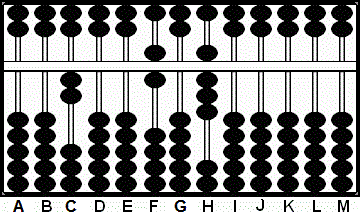Fig. 3 ```Step 3 A B C D E F G H I J K L M       0 0 2 0 0 5 2 8 0 0 0 0 0 forward 1 - 2 Step 3 0 0 2 0 0 6 0 8 0 0 0 0 0 ```

Step 4 and the answer: Compare divisor 2 with 8 on H and follow ==> rule 8 / 2 = forward 4 <== forward 4 to G and subtract 8 from H leaving the answer 64 on rods FG. (Fig.4)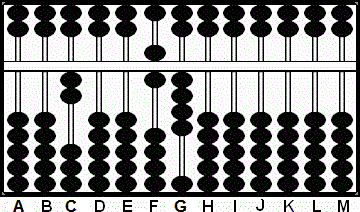Fig. 4 ```Step 3 A B C D E F G H I J K L M       0 0 2 0 0 6 0 8 0 0 0 0 0 forward 4 - 8 Step 4 0 0 2 0 0 6 4 0 0 0 0 0 0 ```

Example 2:  259 ÷ 7 = 37

Step 1: Set the dividend 259 on FGH and the divisor 7 on C.  (Fig.5)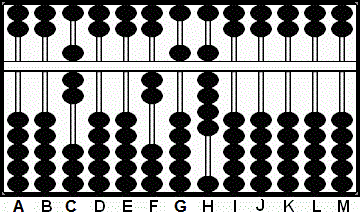Fig. 5 ```Step 1 A B C D E F G H I J K L M       0 0 7 0 0 2 5 9 0 0 0 0 0 ```

Step 2: Compare divisor 7 with 2 on F and follow ==> rule 2 /7 = 2 plus 6 <== The first number in the quotient will be 2. Leave the 2 on F and add 6 to G. With 11 remaining on G we'll have to revise the quotient higher. (Fig.6)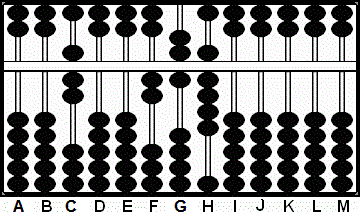Fig. 6 ```Step 2 A B C D E F G H I J K L M       0 0 7 0 0 2 5 9 0 0 0 0 0 (2) plus + 6 Step 2 0 0 7 0 0 2"11"9 0 0 0 0 0``` ` `

Step 3: Revise the quotient: add 1 to F and subtract a further 7 away from G. This leaves interim quotient 3 on F and remainder 49 on GH. (Fig.7)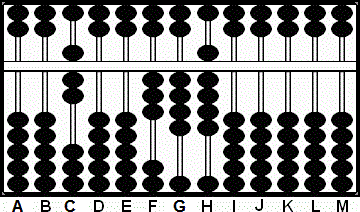Fig. 7 ```Step 3 A B C D E F G H I J K L M       0 0 7 0 0 2"11"9 0 0 0 0 0 revise + 1 - 7 Step 3 0 0 7 0 0 3 4 9 0 0 0 0 0 ```

Step 4: Compare divisor 7 with 4 on G and follow ==> rule 4 /7 = 5  plus 5 <==  The second number in the quotient will be 5.  Remove the 4 from G and replace it with 5. Add 5 to rod H. With 14 remaining on H we'll have to revise the quotient higher. (Fig.8)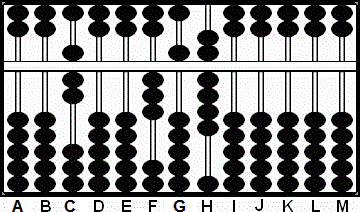Fig. 8 ```Step 4 A B C D E F G H I J K L M       0 0 7 0 0 3 4 9 0 0 0 0 0 (5) plus + 5 Step 4 0 0 7 0 0 3 5"14"0 0 0 0 0 ```

Step 5 and the answer: Revise the quotient and follow ==> rule 14 /7 = forward 2 <== add 2 to 5 on G and subtract a further 14 from H. This leaves the answer 37 on rods FG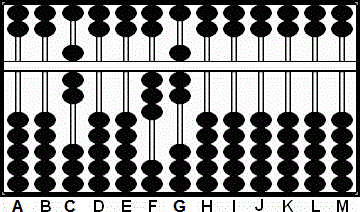Fig. 9 ```Step 5 A B C D E F G H I J K L M       0 0 7 0 0 3 5"14"0 0 0 0 0 Revise + 2 - ("14") Step 5 0 0 7 0 0 3 7 0 0 0 0 0 0 ```

It's evident that some of the above steps could have been done more efficiently. For instance in  Example 2:  Step 3, instead of dividing 7 into the 4 on rod G, we could have looked ahead and noticed 49 on GH. At this point dividing 7 into 49 would have allowed us to come to our answer of 37 that much more quickly. I took the longer approach only because it may be of help to see some of the thinking behind the division table process.

###The Chinese Rule Advantage

Sometimes finding the solution to even the simplest division problem can be a challenge. Often the best way to go about solving a problem is to estimate an answer and try to work it through. But this means the solution needs *thinking* about and that's the trouble. As much as possible the idea behind solving problems on an abacus is to mechanize operations and to minimize mental stress. In fact a good abacus operator can work solving number problems all day long and not feel any mental fatigue at all.

And this is my point. For many of us tackling division problems requires a certain amount of thought, a certain finesse and for some even a certain amount of guess work. If, on the other hand, we attack problems using the Division Table at the top of the page there is no mental stress. And that's an advantage. Just learn the rules and follow them. It's that simple.

Let's move on to three simple examples where there's a two digit divisor.

Here goes....

Example 1: 222 ÷ 37 = 6

This Example uses the rule:  2/3 = 6 plus 2

Step 1: Set up the problem on the suan pan. Choose rod G as the unit rod. Set the divisor 37 on rods AB  and 222 on E - G. (Fig.1)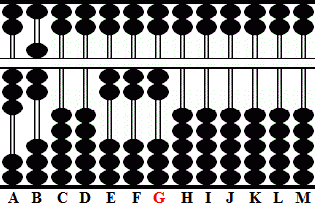Fig. 1 ```Step 1 A B C D E F G H I J K L M       3 7 0 0 2 2 2 0 0 0 0 0 0 ```

Step 2: Compare divisor 3 with 2 on E.  Use the Rule: ==> 2/3 = 6 plus 2 <== Remove the 2 from rod E and replace it with 6. Add 2 to rod F. This leaves the remainder 42 on rods FG.Fig. 2 ```Step 2 A B C D E F G H I J K L M       3 7 0 0 2 2 2 0 0 0 0 0 0 (6) + 2   3 7 0 0 6 4 2 0 0 0 0 0 0 ```

Step 3: Multiply 6 on E by 7 on B and subtract the product 42 from rods FG. Perfect. But I'm not quite finished. We have to identify the new unit rod.
Step 3a and the answer: Because there are two whole numbers in the divisor shift the unit rod two rods to the left. That takes us to rod E. And there it is; the answer 6 on rod E. (Fig. 4)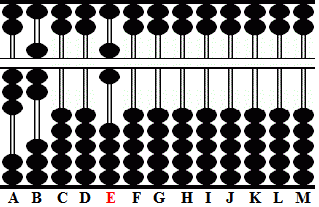Fig. 3 ```Step 3 A B C D E F G H I J K L M       3 7 0 0 6 4 2 0 0 0 0 0 0 - 4 2       3 7 0 0 6 0 0 0 0 0 0 0 0 ```

We all know that when we just follow hard and fast rules it means that we might not always be correct in our first attempt. An answer may very well have to be revised. But that's okay too. The rules are there as a guideline. With their help even complicated problems are easily solved. Just follow the rules and be prepared to revise an answer either higher or lower.

Example 2: 78 ÷ 13 = 6

Use the rule:  7/1 = forward 7. In this example we'll have to revise the answer lower.

Step 1: Set up the problem on the suan pan. Choose rod G as the unit rod. Set the divisor on the left  and the dividend on rods FG. (Fig.1)Fig. 1 ```Step 1 A B C D E F G H I J K L M       1 3 0 0 0 7 8 0 0 0 0 0 0 ```

Step 2: Compare divisor 1 with 7 on rod F. Use Rule: ==> 7/1 = forward 7 <==  Forward 7 from F to rod E.
Step 2a: Multiply 7 on E by 3 on B and subtract 21. With only 08 on rods GH the remainder is too small. Revise the answer lower. (Fig.2)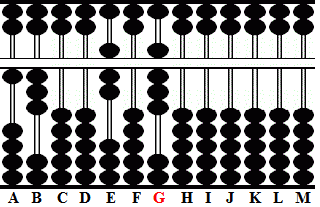Fig. 2 ```Step 2 A B C D E F G H I J K L M       1 3 0 0 7 0 8 0 0 0 0 0 0```

Step 3: Revise the answer lower: subtract 1 from 7 on E to make it 6 then add 1 back to rod F. With 18 on rods FG there is enough to continue. (Fig.3)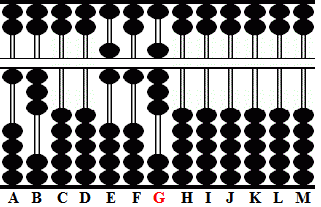Fig. 3 ```Step 3 A B C D E F G H I J K L M       1 3 0 0 7 0 8 0 0 0 0 0 0 revise -1         +1         1 3 0 0 6 1 8 0 0 0 0 0 0 ```

Step 4: Multiply 6 by 3 and subtract the product 18 from rods FG. Perfect. This leaves 6 on rod E with no remainder. Once again identify the new unit rod.
Step 4a and the answer: Because there are two whole numbers in the divisor shift the unit rod two rods to the left. That takes us to rod E. And there it is; the answer 6 on rod E. (Fig. 4)Fig. 4 ```Step 4 A B C D E F G H I J K L M       1 3 0 0 0 1 9 0 0 0 0 0 0         -1 8      1 3 0 0 6 0 0 0 0 0 0 0 0```

Example 3: 192 ÷ 24 = 8

Begin with the rule:  1/5 = 2. In this example we'll have to revise the answer higher.

Step 1: Set the problem. Choose rod H as the unit rod. Set the divisor on the left and the dividend on rods FGH. (Fig. 5)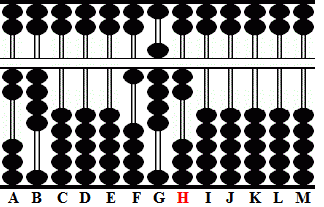Fig. 5 ```Step 1 A B C D E F G H I J K L M       2 4 0 0 0 1 9 2 0 0 0 0 0```

Step 2: Compare 2 with 1 on rod F and use the rule ==> 1/2 = 5 <== Remove the 1 from F and replace it with 5. Multiply 5 x 4 and subtract 20 from rods GH leaving the remainder 72 which is greater than the divisor 24. Revise our answer higher. (Fig. 6)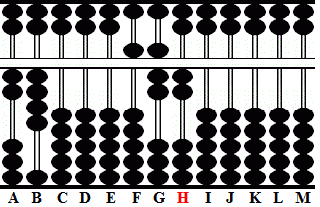Fig. 6 ```Step 2 A B C D E F G H I J K L M       2 4 0 0 0 1 9 2 0 0 0 0 0 (5)         -2 0     2 4 0 0 0 5 7 2 0 0 0 0 0```

Step 3: Revise the answer higher using the rule 7/2 = forward 3. Add 3 to 5 on rod F making it 8. Subtract 6 from G. (Fig.7)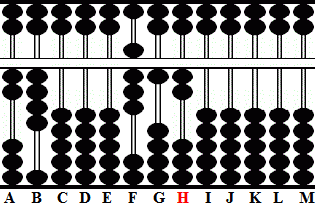Fig. 7 ```Step 3 A B C D E F G H I J K L M       2 4 0 0 0 5 7 2 0 0 0 0 0 revise + 3         -6     2 4 0 0 0 8 1 2 0 0 0 0 0```

Step 3a: Because we've added another 3 to our answer we'll have to multiply 3 x 4 and subtract 12 from rods GH. Identify the new unit rod.
Step 3b and the answer: Because there are two whole numbers in the dividend shift the unit rod two rods to the left. That takes us to rod F. The answer is 8 on rod F. (Fig. 8)Fig. 8 ```Step 3a & 3b A B C D E F G H I J K L M       2 4 0 0 0 8 1 2 0 0 0 0 0         -1 2     2 4 0 0 0 8 0 0 0 0 0 0 0```